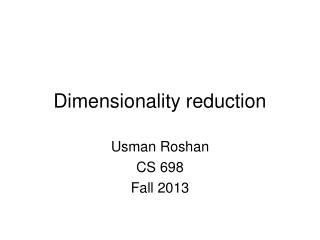# Dimensionality reduction - PowerPoint PPT PresentationDownload PresentationDimensionality reduction

Dimensionality reductionDownload Presentation## Dimensionality reduction

- - - - - - - - - - - - - - - - - - - - - - - - - - - E N D - - - - - - - - - - - - - - - - - - - - - - - - - - -
##### Presentation Transcript

1. Dimensionality reduction Usman Roshan CS 698 Fall 2013

2. Supervised dim reduction: Linear discriminant analysis • Fisher linear discriminant: • Maximize ratio of difference means to sum of variance

3. Linear discriminant analysis • Fisher linear discriminant: • Difference in means of projected data gives us the between-class scatter matrix • Variance gives us within-class scatter matrix

4. Linear discriminant analysis • Fisher linear discriminantsolution: • Take derivative w.r.t. w and set to 0 • This gives us w = cSw-1(m1-m2)

5. Scatter matrices • Sb is between class scatter matrix • Sw is within-class scatter matrix • St= Sb+ Swis total scatter matrix

6. Fisher linear discriminant • General solution is given by eigenvectors of Sw-1Sb

7. Fisher linear discriminant • Problems can happen with calculating the inverse • A different approach is the maximum margin criterion

8. Maximum margin criterion (MMC) • Define the separation between two classes as • S(C) represents the variance of the class. In MMC we use the trace of the scatter matrix to represent the variance. • The scatter matrix is

9. Maximum margin criterion (MMC) • The scatter matrix is • The trace (sum of diagonals) is • Consider an example with two vectors x and y

10. Maximum margin criterion (MMC) • Plug in trace for S(C) and we get • The above can be rewritten as • Where Sw is the within-class scatter matrix • And Sb is the between-class scatter matrix

11. Weighted maximum margin criterion (WMMC) • Adding a weight parameter gives us • In WMMC dimensionality reduction we want to find w that maximizes the above quantity in the projected space. • The solution w is given by the largest eigenvector of the above

12. How to use WMMC for classification? • Reduce dimensionality to fewer features • Run any classification algorithm like nearest means or nearest neighbor. • Experimental results to follow.

13. K-nearest neighbor • Classify a given datapoint to be the majority label of the k closest points • The parameter k is cross-validated • Simple yet can obtain high classification accuracy

14. Weighted maximum variance (WMV) • Find w that maximizes the weighted variance

15. PCA via WMV • Reduces to PCA if Cij = 1/n

16. MMC via WMV • Let yi be class labels and let nk be the size of class k. • Let Gij be 1/n for all i and j and Lij be 1/nk if i and j are in same class. • Then MMC is given by

17. MMC via WMV (proof sketch)

18. Graph Laplacians • We can rewrite WMV with Laplacian matrices. • Recall WMV is • Let L = D – C where Dii = ΣjCij • Then WMV is given by where X = [x1, x2, …, xn] contains each xi as a column. • w is given by largest eigenvector of XLXT

19. Graph Laplacians • Widely used in spectral clustering (see tutorial on course website) • Weights Cij may be obtained via • Epsilon neighborhood graph • K-nearest neighbor graph • Fully connected graph • Allows semi-supervised analysis (where test data is available but not labels)

20. Graph Laplacians • We can perform clustering with the Laplacian • Basic algorithm for k clusters: • Compute first k eigenvectors vi of Laplacian matrix • Let V = [v1, v2, …, vk] • Cluster rows of V (using k-means) • Why does this work?

21. Graph Laplacians • We can cluster data using the mincut problem • Balanced version is NP-hard • We can rewrite balanced mincut problem with graph Laplacians. Still NP-hard because solution is allowed only discrete values • By relaxing to allow real values we obtain spectral clustering.

22. Back to WMV – a two parameter approach • Recall that WMV is given by • Collapse Cij into two parameters • Cij = α < 0 if i and j are in same class • Cij = β > 0 if i and j are in different classes • We call this 2-parameter WMV

23. Experimental results • To evaluate dimensionality reduction for classification we first extract features and then apply 1-nearest neighbor in cross-validation • 20 datasets from UCI machine learning archive • Compare 2PWMV+1NN, WMMC+1NN, PCA+1NN, 1NN • Parameters for 2PWMV+1NN and WMMC+1NN obtained by cross-validation

24. Datasets

25. Results

26. Results

27. Results • Average error: • 2PWMV+1NN: 9.5% (winner in 9 out of 20) • WMMC+1NN: 10% (winner in 7 out of 20) • PCA+1NN: 13.6% • 1NN: 13.8% • Parametric dimensionality reduction does help

28. High dimensional data

29. High dimensional data

30. Results • Average error on high dimensional data: • 2PWMV+1NN: 15.2% • PCA+1NN: 17.8% • 1NN: 22%